# Motion Analysis and Object Tracking¶

## calcOpticalFlowPyrLK¶

Calculates an optical flow for a sparse feature set using the iterative Lucas-Kanade method with pyramids.

C++: void calcOpticalFlowPyrLK(InputArray prevImg, InputArray nextImg, InputArray prevPts, InputOutputArray nextPts, OutputArray status, OutputArray err, Size winSize=Size(21,21), int maxLevel=3, TermCriteria criteria=TermCriteria(TermCriteria::COUNT+TermCriteria::EPS, 30, 0.01), int flags=0, double minEigThreshold=1e-4 )
Python: cv2.calcOpticalFlowPyrLK(prevImg, nextImg, prevPts, nextPts[, status[, err[, winSize[, maxLevel[, criteria[, flags[, minEigThreshold]]]]]]]) → nextPts, status, err
C: void cvCalcOpticalFlowPyrLK(const CvArr* prev, const CvArr* curr, CvArr* prev_pyr, CvArr* curr_pyr, const CvPoint2D32f* prev_features, CvPoint2D32f* curr_features, int count, CvSize win_size, int level, char* status, float* track_error, CvTermCriteria criteria, int flags)
Parameters: prevImg – first 8-bit input image or pyramid constructed by buildOpticalFlowPyramid(). nextImg – second input image or pyramid of the same size and the same type as prevImg. prevPts – vector of 2D points for which the flow needs to be found; point coordinates must be single-precision floating-point numbers. nextPts – output vector of 2D points (with single-precision floating-point coordinates) containing the calculated new positions of input features in the second image; when OPTFLOW_USE_INITIAL_FLOW flag is passed, the vector must have the same size as in the input. status – output status vector (of unsigned chars); each element of the vector is set to 1 if the flow for the corresponding features has been found, otherwise, it is set to 0. err – output vector of errors; each element of the vector is set to an error for the corresponding feature, type of the error measure can be set in flags parameter; if the flow wasn’t found then the error is not defined (use the status parameter to find such cases). winSize – size of the search window at each pyramid level. maxLevel – 0-based maximal pyramid level number; if set to 0, pyramids are not used (single level), if set to 1, two levels are used, and so on; if pyramids are passed to input then algorithm will use as many levels as pyramids have but no more than maxLevel. criteria – parameter, specifying the termination criteria of the iterative search algorithm (after the specified maximum number of iterations criteria.maxCount or when the search window moves by less than criteria.epsilon. flags – operation flags: OPTFLOW_USE_INITIAL_FLOW uses initial estimations, stored in nextPts; if the flag is not set, then prevPts is copied to nextPts and is considered the initial estimate. OPTFLOW_LK_GET_MIN_EIGENVALS use minimum eigen values as an error measure (see minEigThreshold description); if the flag is not set, then L1 distance between patches around the original and a moved point, divided by number of pixels in a window, is used as a error measure. minEigThreshold – the algorithm calculates the minimum eigen value of a 2x2 normal matrix of optical flow equations (this matrix is called a spatial gradient matrix in [Bouguet00]), divided by number of pixels in a window; if this value is less than minEigThreshold, then a corresponding feature is filtered out and its flow is not processed, so it allows to remove bad points and get a performance boost.

The function implements a sparse iterative version of the Lucas-Kanade optical flow in pyramids. See [Bouguet00]. The function is parallelized with the TBB library.

Note

• An example using the Lucas-Kanade optical flow algorithm can be found at opencv_source_code/samples/cpp/lkdemo.cpp
• (Python) An example using the Lucas-Kanade optical flow algorithm can be found at opencv_source_code/samples/python2/lk_track.py
• (Python) An example using the Lucas-Kanade tracker for homography matching can be found at opencv_source_code/samples/python2/lk_homography.py

## buildOpticalFlowPyramid¶

Constructs the image pyramid which can be passed to calcOpticalFlowPyrLK().

C++: int buildOpticalFlowPyramid(InputArray img, OutputArrayOfArrays pyramid, Size winSize, int maxLevel, bool withDerivatives=true, int pyrBorder=BORDER_REFLECT_101, int derivBorder=BORDER_CONSTANT, bool tryReuseInputImage=true)
Python: cv2.buildOpticalFlowPyramid(img, winSize, maxLevel[, pyramid[, withDerivatives[, pyrBorder[, derivBorder[, tryReuseInputImage]]]]]) → retval, pyramid
Parameters: img – 8-bit input image. pyramid – output pyramid. winSize – window size of optical flow algorithm. Must be not less than winSize argument of calcOpticalFlowPyrLK(). It is needed to calculate required padding for pyramid levels. maxLevel – 0-based maximal pyramid level number. withDerivatives – set to precompute gradients for the every pyramid level. If pyramid is constructed without the gradients then calcOpticalFlowPyrLK() will calculate them internally. pyrBorder – the border mode for pyramid layers. derivBorder – the border mode for gradients. tryReuseInputImage – put ROI of input image into the pyramid if possible. You can pass false to force data copying. number of levels in constructed pyramid. Can be less than maxLevel.

## calcOpticalFlowFarneback¶

Computes a dense optical flow using the Gunnar Farneback’s algorithm.

C++: void calcOpticalFlowFarneback(InputArray prev, InputArray next, InputOutputArray flow, double pyr_scale, int levels, int winsize, int iterations, int poly_n, double poly_sigma, int flags)
C: void cvCalcOpticalFlowFarneback(const CvArr* prev, const CvArr* next, CvArr* flow, double pyr_scale, int levels, int winsize, int iterations, int poly_n, double poly_sigma, int flags)
Python: cv2.calcOpticalFlowFarneback(prev, next, flow, pyr_scale, levels, winsize, iterations, poly_n, poly_sigma, flags) → flow
Parameters: prev – first 8-bit single-channel input image. next – second input image of the same size and the same type as prev. flow – computed flow image that has the same size as prev and type CV_32FC2. pyr_scale – parameter, specifying the image scale (<1) to build pyramids for each image; pyr_scale=0.5 means a classical pyramid, where each next layer is twice smaller than the previous one. levels – number of pyramid layers including the initial image; levels=1 means that no extra layers are created and only the original images are used. winsize – averaging window size; larger values increase the algorithm robustness to image noise and give more chances for fast motion detection, but yield more blurred motion field. iterations – number of iterations the algorithm does at each pyramid level. poly_n – size of the pixel neighborhood used to find polynomial expansion in each pixel; larger values mean that the image will be approximated with smoother surfaces, yielding more robust algorithm and more blurred motion field, typically poly_n =5 or 7. poly_sigma – standard deviation of the Gaussian that is used to smooth derivatives used as a basis for the polynomial expansion; for poly_n=5, you can set poly_sigma=1.1, for poly_n=7, a good value would be poly_sigma=1.5. flags – operation flags that can be a combination of the following: OPTFLOW_USE_INITIAL_FLOW uses the input flow as an initial flow approximation. OPTFLOW_FARNEBACK_GAUSSIAN uses the Gaussian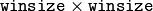filter instead of a box filter of the same size for optical flow estimation; usually, this option gives z more accurate flow than with a box filter, at the cost of lower speed; normally, winsize for a Gaussian window should be set to a larger value to achieve the same level of robustness.

The function finds an optical flow for each prev pixel using the [Farneback2003] algorithm so that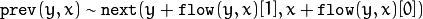Note

• An example using the optical flow algorithm described by Gunnar Farneback can be found at opencv_source_code/samples/cpp/fback.cpp
• (Python) An example using the optical flow algorithm described by Gunnar Farneback can be found at opencv_source_code/samples/python2/opt_flow.py

## estimateRigidTransform¶

Computes an optimal affine transformation between two 2D point sets.

C++: Mat estimateRigidTransform(InputArray src, InputArray dst, bool fullAffine)
Python: cv2.estimateRigidTransform(src, dst, fullAffine) → retval
Parameters: src – First input 2D point set stored in std::vector or Mat, or an image stored in Mat. dst – Second input 2D point set of the same size and the same type as A, or another image. fullAffine – If true, the function finds an optimal affine transformation with no additional restrictions (6 degrees of freedom). Otherwise, the class of transformations to choose from is limited to combinations of translation, rotation, and uniform scaling (5 degrees of freedom).

The function finds an optimal affine transform [A|b] (a 2 x 3 floating-point matrix) that approximates best the affine transformation between:

• Two point sets
• Two raster images. In this case, the function first finds some features in the src image and finds the corresponding features in dst image. After that, the problem is reduced to the first case.

In case of point sets, the problem is formulated as follows: you need to find a 2x2 matrix A and 2x1 vector b so that: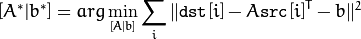where src[i] and dst[i] are the i-th points in src and dst, respectively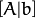can be either arbitrary (when fullAffine=true ) or have a form of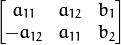when fullAffine=false .

## findTransformECC¶

Finds the geometric transform (warp) between two images in terms of the ECC criterion [EP08].

C++: double findTransformECC(InputArray templateImage, InputArray inputImage, InputOutputArray warpMatrix, int motionType=MOTION_AFFINE, TermCriteria criteria=TermCriteria(TermCriteria::COUNT+TermCriteria::EPS, 50, 0.001))
Python: cv2.findTransformECC(templateImage, inputImage, warpMatrix[, motionType[, criteria]]) → retval, warpMatrix
Parameters: templateImage – single-channel template image; CV_8U or CV_32F array. inputImage – single-channel input image which should be warped with the final warpMatrix in order to provide an image similar to templateImage, same type as temlateImage. warpMatrix – floating-point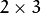or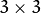mapping matrix (warp). motionType – parameter, specifying the type of motion: MOTION_TRANSLATION sets a translational motion model; warpMatrix iswith the first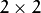part being the unity matrix and the rest two parameters being estimated. MOTION_EUCLIDEAN sets a Euclidean (rigid) transformation as motion model; three parameters are estimated; warpMatrix is. MOTION_AFFINE sets an affine motion model (DEFAULT); six parameters are estimated; warpMatrix is. MOTION_HOMOGRAPHY sets a homography as a motion model; eight parameters are estimated;warpMatrix is. criteria – parameter, specifying the termination criteria of the ECC algorithm; criteria.epsilon defines the threshold of the increment in the correlation coefficient between two iterations (a negative criteria.epsilon makes criteria.maxcount the only termination criterion). Default values are shown in the declaration above.

The function estimates the optimum transformation (warpMatrix) with respect to ECC criterion ([EP08]), that is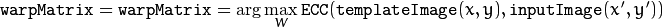where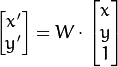(the equation holds with homogeneous coordinates for homography). It returns the final enhanced correlation coefficient, that is the correlation coefficient between the template image and the final warped input image. When amatrix is given with motionType =0, 1 or 2, the third row is ignored.

Unlike findHomography() and estimateRigidTransform(), the function findTransformECC() implements an area-based alignment that builds on intensity similarities. In essence, the function updates the initial transformation that roughly aligns the images. If this information is missing, the identity warp (unity matrix) should be given as input. Note that if images undergo strong displacements/rotations, an initial transformation that roughly aligns the images is necessary (e.g., a simple euclidean/similarity transform that allows for the images showing the same image content approximately). Use inverse warping in the second image to take an image close to the first one, i.e. use the flag WARP_INVERSE_MAP with warpAffine() or warpPerspective(). See also the OpenCV sample image_alignment.cpp that demonstrates the use of the function. Note that the function throws an exception if algorithm does not converges.

See also

## CamShift¶

Finds an object center, size, and orientation.

C++: RotatedRect CamShift(InputArray probImage, Rect& window, TermCriteria criteria)
Python: cv2.CamShift(probImage, window, criteria) → retval, window
C: int cvCamShift(const CvArr* prob_image, CvRect window, CvTermCriteria criteria, CvConnectedComp* comp, CvBox2D* box=NULL )
Parameters: probImage – Back projection of the object histogram. See calcBackProject() . window – Initial search window. criteria – Stop criteria for the underlying meanShift() . (in old interfaces) Number of iterations CAMSHIFT took to converge

The function implements the CAMSHIFT object tracking algorithm [Bradski98]. First, it finds an object center using meanShift() and then adjusts the window size and finds the optimal rotation. The function returns the rotated rectangle structure that includes the object position, size, and orientation. The next position of the search window can be obtained with RotatedRect::boundingRect() .

See the OpenCV sample camshiftdemo.c that tracks colored objects.

Note

• (Python) A sample explaining the camshift tracking algorithm can be found at opencv_source_code/samples/python2/camshift.py

## meanShift¶

Finds an object on a back projection image.

C++: int meanShift(InputArray probImage, Rect& window, TermCriteria criteria)
Python: cv2.meanShift(probImage, window, criteria) → retval, window
C: int cvMeanShift(const CvArr* prob_image, CvRect window, CvTermCriteria criteria, CvConnectedComp* comp)
Parameters: probImage – Back projection of the object histogram. See calcBackProject() for details. window – Initial search window. criteria – Stop criteria for the iterative search algorithm. Number of iterations CAMSHIFT took to converge.

The function implements the iterative object search algorithm. It takes the input back projection of an object and the initial position. The mass center in window of the back projection image is computed and the search window center shifts to the mass center. The procedure is repeated until the specified number of iterations criteria.maxCount is done or until the window center shifts by less than criteria.epsilon . The algorithm is used inside CamShift() and, unlike CamShift() , the search window size or orientation do not change during the search. You can simply pass the output of calcBackProject() to this function. But better results can be obtained if you pre-filter the back projection and remove the noise. For example, you can do this by retrieving connected components with findContours() , throwing away contours with small area ( contourArea() ), and rendering the remaining contours with drawContours() .

Note

• A mean-shift tracking sample can be found at opencv_source_code/samples/cpp/camshiftdemo.cpp

## KalmanFilter¶

class KalmanFilter

Kalman filter class.

The class implements a standard Kalman filter http://en.wikipedia.org/wiki/Kalman_filter, [Welch95]. However, you can modify transitionMatrix, controlMatrix, and measurementMatrix to get an extended Kalman filter functionality. See the OpenCV sample kalman.cpp .

Note

• An example using the standard Kalman filter can be found at opencv_source_code/samples/cpp/kalman.cpp

## KalmanFilter::KalmanFilter¶

The constructors.

C++: KalmanFilter::KalmanFilter()
C++: KalmanFilter::KalmanFilter(int dynamParams, int measureParams, int controlParams=0, int type=CV_32F)
Python: cv2.KalmanFilter([dynamParams, measureParams[, controlParams[, type]]]) → <KalmanFilter object>
C: CvKalman* cvCreateKalman(int dynam_params, int measure_params, int control_params=0 )

The full constructor.

Parameters: dynamParams – Dimensionality of the state. measureParams – Dimensionality of the measurement. controlParams – Dimensionality of the control vector. type – Type of the created matrices that should be CV_32F or CV_64F.

Note

In C API when CvKalman* kalmanFilter structure is not needed anymore, it should be released with cvReleaseKalman(&kalmanFilter)

## KalmanFilter::init¶

Re-initializes Kalman filter. The previous content is destroyed.

C++: void KalmanFilter::init(int dynamParams, int measureParams, int controlParams=0, int type=CV_32F)
Parameters: dynamParams – Dimensionalityensionality of the state. measureParams – Dimensionality of the measurement. controlParams – Dimensionality of the control vector. type – Type of the created matrices that should be CV_32F or CV_64F.

## KalmanFilter::predict¶

Computes a predicted state.

C++: const Mat& KalmanFilter::predict(const Mat& control=Mat())
Python: cv2.KalmanFilter.predict([control]) → retval
C: const CvMat* cvKalmanPredict(CvKalman* kalman, const CvMat* control=NULL)
Parameters: control – The optional input control

## KalmanFilter::correct¶

Updates the predicted state from the measurement.

C++: const Mat& KalmanFilter::correct(const Mat& measurement)
Python: cv2.KalmanFilter.correct(measurement) → retval
C: const CvMat* cvKalmanCorrect(CvKalman* kalman, const CvMat* measurement)
Parameters: measurement – The measured system parameters

## BackgroundSubtractor¶

class BackgroundSubtractor : public Algorithm

Base class for background/foreground segmentation.

class BackgroundSubtractor : public Algorithm
{
public:
virtual ~BackgroundSubtractor();
virtual void apply(InputArray image, OutputArray fgmask, double learningRate=0);
virtual void getBackgroundImage(OutputArray backgroundImage) const;
};


The class is only used to define the common interface for the whole family of background/foreground segmentation algorithms.

## BackgroundSubtractor::apply¶

Computes a foreground mask.

C++: void BackgroundSubtractor::apply(InputArray image, OutputArray fgmask, double learningRate=-1)
Python: cv2.BackgroundSubtractor.apply(image[, fgmask[, learningRate]]) → fgmask
Parameters: image – Next video frame. fgmask – The output foreground mask as an 8-bit binary image. learningRate – The value between 0 and 1 that indicates how fast the background model is learnt. Negative parameter value makes the algorithm to use some automatically chosen learning rate. 0 means that the background model is not updated at all, 1 means that the background model is completely reinitialized from the last frame.

## BackgroundSubtractor::getBackgroundImage¶

Computes a background image.

C++: void BackgroundSubtractor::getBackgroundImage(OutputArray backgroundImage) const
Parameters: backgroundImage – The output background image.

Note

Sometimes the background image can be very blurry, as it contain the average background statistics.

## BackgroundSubtractorMOG¶

class BackgroundSubtractorMOG : public BackgroundSubtractor

Gaussian Mixture-based Background/Foreground Segmentation Algorithm.

The class implements the algorithm described in [KB2001].

## createBackgroundSubtractorMOG¶

Creates mixture-of-gaussian background subtractor

C++: Ptr<BackgroundSubtractorMOG> createBackgroundSubtractorMOG(int history=200, int nmixtures=5, double backgroundRatio=0.7, double noiseSigma=0)
Python: cv2.createBackgroundSubtractorMOG([history[, nmixtures[, backgroundRatio[, noiseSigma]]]]) → retval
Parameters: history – Length of the history. nmixtures – Number of Gaussian mixtures. backgroundRatio – Background ratio. noiseSigma – Noise strength (standard deviation of the brightness or each color channel). 0 means some automatic value.

## BackgroundSubtractorMOG2¶

Gaussian Mixture-based Background/Foreground Segmentation Algorithm.

class BackgroundSubtractorMOG2 : public BackgroundSubtractor

The class implements the Gaussian mixture model background subtraction described in [Zivkovic2004] and [Zivkovic2006] .

## createBackgroundSubtractorMOG2¶

Creates MOG2 Background Subtractor

C++: Ptr<BackgroundSubtractorMOG2> createBackgroundSubtractorMOG2(int history=500, double varThreshold=16, bool detectShadows=true )
Parameters: history – Length of the history. varThreshold – Threshold on the squared Mahalanobis distance between the pixel and the model to decide whether a pixel is well described by the background model. This parameter does not affect the background update. detectShadows – If true, the algorithm will detect shadows and mark them. It decreases the speed a bit, so if you do not need this feature, set the parameter to false.

## BackgroundSubtractorMOG2::getHistory¶

Returns the number of last frames that affect the background model

C++: int BackgroundSubtractorMOG2::getHistory() const

## BackgroundSubtractorMOG2::setHistory¶

Sets the number of last frames that affect the background model

C++: void BackgroundSubtractorMOG2::setHistory(int history)

## BackgroundSubtractorMOG2::getNMixtures¶

Returns the number of gaussian components in the background model

C++: int BackgroundSubtractorMOG2::getNMixtures() const

## BackgroundSubtractorMOG2::setNMixtures¶

Sets the number of gaussian components in the background model. The model needs to be reinitalized to reserve memory.

C++: void BackgroundSubtractorMOG2::setNMixtures(int nmixtures)

## BackgroundSubtractorMOG2::getBackgroundRatio¶

Returns the “background ratio” parameter of the algorithm

C++: double BackgroundSubtractorMOG2::getBackgroundRatio() const

If a foreground pixel keeps semi-constant value for about backgroundRatio*history frames, it’s considered background and added to the model as a center of a new component. It corresponds to TB parameter in the paper.

## BackgroundSubtractorMOG2::setBackgroundRatio¶

Sets the “background ratio” parameter of the algorithm

C++: void BackgroundSubtractorMOG2::setBackgroundRatio(double ratio)

## BackgroundSubtractorMOG2::getVarThreshold¶

Returns the variance threshold for the pixel-model match

C++: double BackgroundSubtractorMOG2::getVarThreshold() const

The main threshold on the squared Mahalanobis distance to decide if the sample is well described by the background model or not. Related to Cthr from the paper.

## BackgroundSubtractorMOG2::setVarThreshold¶

Sets the variance threshold for the pixel-model match

C++: void BackgroundSubtractorMOG2::setVarThreshold(double varThreshold)

## BackgroundSubtractorMOG2::getVarThresholdGen¶

Returns the variance threshold for the pixel-model match used for new mixture component generation

C++: double BackgroundSubtractorMOG2::getVarThresholdGen() const

Threshold for the squared Mahalanobis distance that helps decide when a sample is close to the existing components (corresponds to Tg in the paper). If a pixel is not close to any component, it is considered foreground or added as a new component. 3 sigma => Tg=3*3=9 is default. A smaller Tg value generates more components. A higher Tg value may result in a small number of components but they can grow too large.

## BackgroundSubtractorMOG2::setVarThresholdGen¶

Sets the variance threshold for the pixel-model match used for new mixture component generation

C++: void BackgroundSubtractorMOG2::setVarThresholdGen(double varThresholdGen)

## BackgroundSubtractorMOG2::getVarInit¶

Returns the initial variance of each gaussian component

C++: double BackgroundSubtractorMOG2::getVarInit() const

## BackgroundSubtractorMOG2::setVarInit¶

Sets the initial variance of each gaussian component

C++: void BackgroundSubtractorMOG2::setVarInit(double varInit)

## BackgroundSubtractorMOG2::getComplexityReductionThreshold¶

Returns the complexity reduction threshold

C++: double BackgroundSubtractorMOG2::getComplexityReductionThreshold() const

This parameter defines the number of samples needed to accept to prove the component exists. CT=0.05 is a default value for all the samples. By setting CT=0 you get an algorithm very similar to the standard Stauffer&Grimson algorithm.

## BackgroundSubtractorMOG2::setComplexityReductionThreshold¶

Sets the complexity reduction threshold

C++: void BackgroundSubtractorMOG2::setComplexityReductionThreshold(double ct)

## BackgroundSubtractorMOG2::getDetectShadows¶

Returns the shadow detection flag

C++: bool BackgroundSubtractorMOG2::getDetectShadows() const

If true, the algorithm detects shadows and marks them. See createBackgroundSubtractorMOG2 for details.

## BackgroundSubtractorMOG2::setDetectShadows¶

Enables or disables shadow detection

C++: void BackgroundSubtractorMOG2::setDetectShadows(bool detectShadows)

## BackgroundSubtractorMOG2::getShadowValue¶

Returns the shadow value

C++: int BackgroundSubtractorMOG2::getShadowValue() const

Shadow value is the value used to mark shadows in the foreground mask. Default value is 127. Value 0 in the mask always means background, 255 means foreground.

## BackgroundSubtractorMOG2::setShadowValue¶

Sets the shadow value

C++: void BackgroundSubtractorMOG2::setShadowValue(int value)

## BackgroundSubtractorMOG2::getShadowThreshold¶

Returns the shadow threshold

C++: double BackgroundSubtractorMOG2::getShadowThreshold() const

A shadow is detected if pixel is a darker version of the background. The shadow threshold (Tau in the paper) is a threshold defining how much darker the shadow can be. Tau= 0.5 means that if a pixel is more than twice darker then it is not shadow. See Prati, Mikic, Trivedi and Cucchiarra, Detecting Moving Shadows..., IEEE PAMI,2003.

## BackgroundSubtractorMOG2::setShadowThreshold¶

Sets the shadow threshold

C++: void BackgroundSubtractorMOG2::setShadowThreshold(double threshold)

## BackgroundSubtractorKNN¶

K-nearest neigbours - based Background/Foreground Segmentation Algorithm.

class BackgroundSubtractorKNN : public BackgroundSubtractor

The class implements the K-nearest neigbours background subtraction described in [Zivkovic2006] . Very efficient if number of foreground pixels is low.

## createBackgroundSubtractorKNN¶

Creates KNN Background Subtractor

C++: Ptr<BackgroundSubtractorKNN> createBackgroundSubtractorKNN(int history=500, double dist2Threshold=400.0, bool detectShadows=true )
Parameters: history – Length of the history. dist2Threshold – Threshold on the squared distance between the pixel and the sample to decide whether a pixel is close to that sample. This parameter does not affect the background update. detectShadows – If true, the algorithm will detect shadows and mark them. It decreases the speed a bit, so if you do not need this feature, set the parameter to false.

## BackgroundSubtractorKNN::getHistory¶

Returns the number of last frames that affect the background model

C++: int BackgroundSubtractorKNN::getHistory() const

## BackgroundSubtractorKNN::setHistory¶

Sets the number of last frames that affect the background model

C++: void BackgroundSubtractorKNN::setHistory(int history)

## BackgroundSubtractorKNN::getNSamples¶

Returns the number of data samples in the background model

C++: int BackgroundSubtractorKNN::getNSamples() const

## BackgroundSubtractorKNN::setNSamples¶

Sets the number of data samples in the background model. The model needs to be reinitalized to reserve memory.

C++: void BackgroundSubtractorKNN::setNSamples(int _nN)

## BackgroundSubtractorKNN::getDist2Threshold¶

Returns the threshold on the squared distance between the pixel and the sample

C++: double BackgroundSubtractorKNN::getDist2Threshold() const

The threshold on the squared distance between the pixel and the sample to decide whether a pixel is close to a data sample.

## BackgroundSubtractorKNN::setDist2Threshold¶

Sets the threshold on the squared distance

C++: void BackgroundSubtractorKNN::setDist2Threshold(double _dist2Threshold)

## BackgroundSubtractorKNN::getkNNSamples¶

Returns the number of neighbours, the k in the kNN. K is the number of samples that need to be within dist2Threshold in order to decide that that pixel is matching the kNN background model.

C++: int BackgroundSubtractorKNN::getkNNSamples() const

## BackgroundSubtractorKNN::setkNNSamples¶

Sets the k in the kNN. How many nearest neigbours need to match.

C++: void BackgroundSubtractorKNN::setkNNSamples(int _nkNN)

## BackgroundSubtractorKNN::getDetectShadows¶

Returns the shadow detection flag

C++: bool BackgroundSubtractorKNN::getDetectShadows() const

If true, the algorithm detects shadows and marks them. See createBackgroundSubtractorKNN for details.

## BackgroundSubtractorKNN::setDetectShadows¶

Enables or disables shadow detection

C++: void BackgroundSubtractorKNN::setDetectShadows(bool detectShadows)

## BackgroundSubtractorKNN::getShadowValue¶

Returns the shadow value

C++: int BackgroundSubtractorKNN::getShadowValue() const

Shadow value is the value used to mark shadows in the foreground mask. Default value is 127. Value 0 in the mask always means background, 255 means foreground.

## BackgroundSubtractorKNN::setShadowValue¶

Sets the shadow value

C++: void BackgroundSubtractorKNN::setShadowValue(int value)

## BackgroundSubtractorKNN::getShadowThreshold¶

Returns the shadow threshold

C++: double BackgroundSubtractorKNN::getShadowThreshold() const

A shadow is detected if pixel is a darker version of the background. The shadow threshold (Tau in the paper) is a threshold defining how much darker the shadow can be. Tau= 0.5 means that if a pixel is more than twice darker then it is not shadow. See Prati, Mikic, Trivedi and Cucchiarra, Detecting Moving Shadows..., IEEE PAMI,2003.

## BackgroundSubtractorKNN::setShadowThreshold¶

Sets the shadow threshold

C++: void BackgroundSubtractorKNN::setShadowThreshold(double threshold)

## BackgroundSubtractorGMG¶

Background Subtractor module based on the algorithm given in [Gold2012].

class BackgroundSubtractorGMG : public BackgroundSubtractor

## createBackgroundSubtractorGMG¶

Creates a GMG Background Subtractor

C++: Ptr<BackgroundSubtractorGMG> createBackgroundSubtractorGMG(int initializationFrames=120, double decisionThreshold=0.8)
Python: cv2.createBackgroundSubtractorGMG([initializationFrames[, decisionThreshold]]) → retval
Parameters: initializationFrames – number of frames used to initialize the background models. decisionThreshold – Threshold value, above which it is marked foreground, else background.

## BackgroundSubtractorGMG::getNumFrames¶

Returns the number of frames used to initialize background model.

C++: int BackgroundSubtractorGMG::getNumFrames() const

## BackgroundSubtractorGMG::setNumFrames¶

Sets the number of frames used to initialize background model.

C++: void BackgroundSubtractorGMG::setNumFrames(int nframes)

## BackgroundSubtractorGMG::getDefaultLearningRate¶

Returns the learning rate of the algorithm. It lies between 0.0 and 1.0. It determines how quickly features are “forgotten” from histograms.

C++: double BackgroundSubtractorGMG::getDefaultLearningRate() const

## BackgroundSubtractorGMG::setDefaultLearningRate¶

Sets the learning rate of the algorithm.

C++: void BackgroundSubtractorGMG::setDefaultLearningRate(double lr)

## BackgroundSubtractorGMG::getDecisionThreshold¶

Returns the value of decision threshold. Decision value is the value above which pixel is determined to be FG.

C++: double BackgroundSubtractorGMG::getDecisionThreshold() const

## BackgroundSubtractorGMG::setDecisionThreshold¶

Sets the value of decision threshold.

C++: void BackgroundSubtractorGMG::setDecisionThreshold(double thresh)

## BackgroundSubtractorGMG::getMaxFeatures¶

Returns total number of distinct colors to maintain in histogram.

C++: int BackgroundSubtractorGMG::getMaxFeatures() const

## BackgroundSubtractorGMG::setMaxFeatures¶

Sets total number of distinct colors to maintain in histogram.

C++: void BackgroundSubtractorGMG::setMaxFeatures(int maxFeatures)

## BackgroundSubtractorGMG::getQuantizationLevels¶

Returns the parameter used for quantization of color-space. It is the number of discrete levels in each channel to be used in histograms.

C++: int BackgroundSubtractorGMG::getQuantizationLevels() const

## BackgroundSubtractorGMG::setQuantizationLevels¶

Sets the parameter used for quantization of color-space

C++: void BackgroundSubtractorGMG::setQuantizationLevels(int nlevels)

## BackgroundSubtractorGMG::getSmoothingRadius¶

Returns the kernel radius used for morphological operations

C++: int BackgroundSubtractorGMG::getSmoothingRadius() const

## BackgroundSubtractorGMG::setSmoothingRadius¶

Sets the kernel radius used for morphological operations

C++: void BackgroundSubtractorGMG::setSmoothingRadius(int radius)

## BackgroundSubtractorGMG::getUpdateBackgroundModel¶

Returns the status of background model update

C++: bool BackgroundSubtractorGMG::getUpdateBackgroundModel() const

## BackgroundSubtractorGMG::setUpdateBackgroundModel¶

Sets the status of background model update

C++: void BackgroundSubtractorGMG::setUpdateBackgroundModel(bool update)

## BackgroundSubtractorGMG::getMinVal¶

Returns the minimum value taken on by pixels in image sequence. Usually 0.

C++: double BackgroundSubtractorGMG::getMinVal() const

## BackgroundSubtractorGMG::setMinVal¶

Sets the minimum value taken on by pixels in image sequence.

C++: void BackgroundSubtractorGMG::setMinVal(double val)

## BackgroundSubtractorGMG::getMaxVal¶

Returns the maximum value taken on by pixels in image sequence. e.g. 1.0 or 255.

C++: double BackgroundSubtractorGMG::getMaxVal() const

## BackgroundSubtractorGMG::setMaxVal¶

Sets the maximum value taken on by pixels in image sequence.

C++: void BackgroundSubtractorGMG::setMaxVal(double val)

## BackgroundSubtractorGMG::getBackgroundPrior¶

Returns the prior probability that each individual pixel is a background pixel.

C++: double BackgroundSubtractorGMG::getBackgroundPrior() const

## BackgroundSubtractorGMG::setBackgroundPrior¶

Sets the prior probability that each individual pixel is a background pixel.

C++: void BackgroundSubtractorGMG::setBackgroundPrior(double bgprior)

## createOptFlow_DualTVL1¶

“Dual TV L1” Optical Flow Algorithm.

C++: Ptr<DenseOpticalFlow> createOptFlow_DualTVL1()

The class implements the “Dual TV L1” optical flow algorithm described in [Zach2007] and [Javier2012] .

Here are important members of the class that control the algorithm, which you can set after constructing the class instance:

double tau

Time step of the numerical scheme.

double lambda

Weight parameter for the data term, attachment parameter. This is the most relevant parameter, which determines the smoothness of the output. The smaller this parameter is, the smoother the solutions we obtain. It depends on the range of motions of the images, so its value should be adapted to each image sequence.

double theta

Weight parameter for (u - v)^2, tightness parameter. It serves as a link between the attachment and the regularization terms. In theory, it should have a small value in order to maintain both parts in correspondence. The method is stable for a large range of values of this parameter.

int nscales

Number of scales used to create the pyramid of images.

int warps

Number of warpings per scale. Represents the number of times that I1(x+u0) and grad( I1(x+u0) ) are computed per scale. This is a parameter that assures the stability of the method. It also affects the running time, so it is a compromise between speed and accuracy.

double epsilon

Stopping criterion threshold used in the numerical scheme, which is a trade-off between precision and running time. A small value will yield more accurate solutions at the expense of a slower convergence.

int iterations

Stopping criterion iterations number used in the numerical scheme.

## DenseOpticalFlow::calc¶

Calculates an optical flow.

C++: void DenseOpticalFlow::calc(InputArray I0, InputArray I1, InputOutputArray flow)
Parameters: I0 – first 8-bit single-channel input image. I1 – second input image of the same size and the same type as I0 . flow – computed flow image that has the same size as I0 and type CV_32FC2 .

## DenseOpticalFlow::collectGarbage¶

Releases all inner buffers.

C++: void DenseOpticalFlow::collectGarbage()
 [Bouguet00] (1, 2) Jean-Yves Bouguet. Pyramidal Implementation of the Lucas Kanade Feature Tracker.
 [Bradski98] Bradski, G.R. “Computer Vision Face Tracking for Use in a Perceptual User Interface”, Intel, 1998
 [EP08] (1, 2) Evangelidis, G.D. and Psarakis E.Z. “Parametric Image Alignment using Enhanced Correlation Coefficient Maximization”, IEEE Transactions on PAMI, vol. 32, no. 10, 2008
 [Farneback2003] Gunnar Farneback, Two-frame motion estimation based on polynomial expansion, Lecture Notes in Computer Science, 2003, (2749), , 363-370.
 [Horn81] Berthold K.P. Horn and Brian G. Schunck. Determining Optical Flow. Artificial Intelligence, 17, pp. 185-203, 1981.
 [KB2001] KadewTraKuPong and R. Bowden. “An improved adaptive background mixture model for real-time tracking with shadow detection”, Proc. 2nd European Workshop on Advanced Video-Based Surveillance Systems, 2001: http://personal.ee.surrey.ac.uk/Personal/R.Bowden/publications/avbs01/avbs01.pdf
 [Javier2012] Javier Sanchez, Enric Meinhardt-Llopis and Gabriele Facciolo. “TV-L1 Optical Flow Estimation”.
 [Lucas81] Lucas, B., and Kanade, T. An Iterative Image Registration Technique with an Application to Stereo Vision, Proc. of 7th International Joint Conference on Artificial Intelligence (IJCAI), pp. 674-679.
 [Welch95] Greg Welch and Gary Bishop “An Introduction to the Kalman Filter”, 1995
 [Zach2007] Zach, T. Pock and H. Bischof. “A Duality Based Approach for Realtime TV-L1 Optical Flow”, In Proceedings of Pattern Recognition (DAGM), Heidelberg, Germany, pp. 214-223, 2007
 [Zivkovic2004] Zivkovic. “Improved adaptive Gausian mixture model for background subtraction”, International Conference Pattern Recognition, UK, August, 2004, http://www.zoranz.net/Publications/zivkovic2004ICPR.pdf. The code is very fast and performs also shadow detection. Number of Gausssian components is adapted per pixel.
 [Zivkovic2006] (1, 2) Z.Zivkovic, F. van der Heijden. “Efficient Adaptive Density Estimation per Image Pixel for the Task of Background Subtraction”, Pattern Recognition Letters, vol. 27, no. 7, pages 773-780, 2006.
 [Gold2012] Andrew B. Godbehere, Akihiro Matsukawa, Ken Goldberg, “Visual Tracking of Human Visitors under Variable-Lighting Conditions for a Responsive Audio Art Installation”, American Control Conference, Montreal, June 2012.